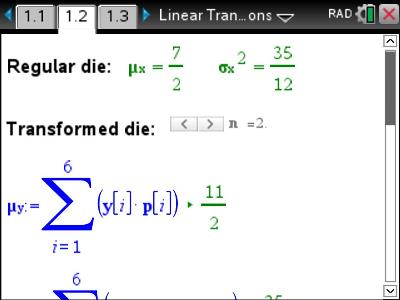# Activities

•• ##### Subject Area

• Standard: VCE: Specialist Mathematics: Specialist Mathematics

• ##### AuthorAust Senior

30 Minutes

• ##### Device
• TI-Nspire™ CAS
• ##### Software

TI-Nspire™ CAS
TI-Nspire™ CAS Navigator™ NC System

4.2

## Linear Transformations of a Random Variable#### Activity Overview

This activity presents an intuitive approach to Linear Transformations of a random variable. If your students don’t understand E(aX+b) or Var(aX+b) then this is a good place to start. By building on a simple familiar example students can validate their intuition using the tables, graphs, spreadsheets and notation to build an better understanding of E(aX+b) and Var(aX+b).

#### Objectives

Understand linear combinations of random variables including:

• E(aX+b)=a.E(X)+b
• Var(aX+b)=a2.Var(X)

#### Vocabulary

Expected value, mean, variance, standard deviation, random variable, translation, transformation